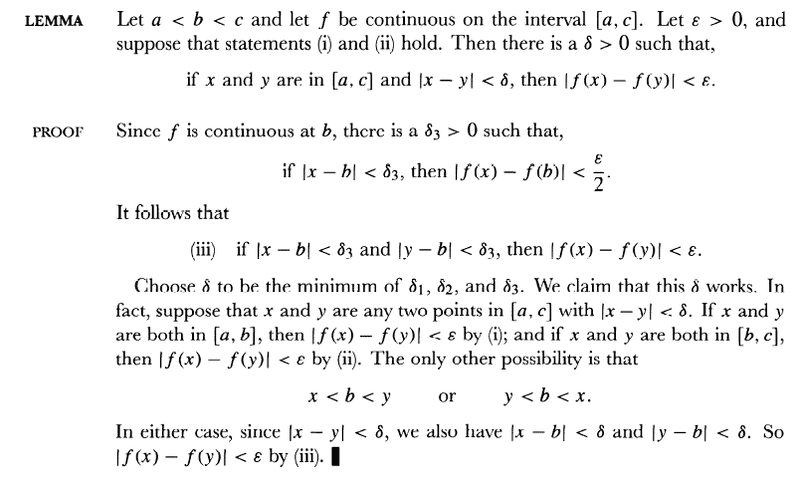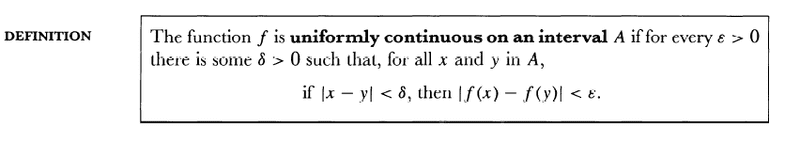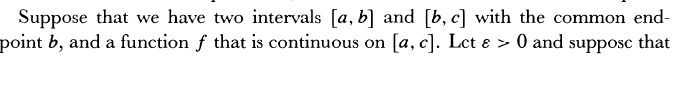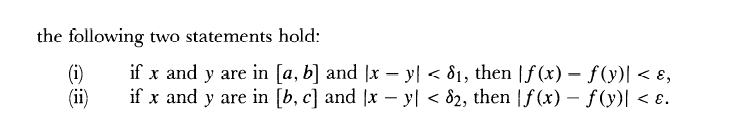# Understanding uniform continuity....

• I
• Alpharup
In summary, the continuity of function f at b is proved by proving that for every ε>0, there exists a δ>0 such that for all x and y satisfying |x-y|<δ3, implies |f(x)-f(y)|<ε.f

#### Alpharup

Let us have a continuous function f which is uniformly continuous on [a,b] and [b,c]...
Then Spivak says, f is uniformly continuous on [a,c]...
For prving this, he invokes the continuity of f on b...
My questions here are:
1.For a given ε, we have a δ1 which works on whole of interval [a,b] and similarly, for this ε, δ2 works for all of [b,c]...Why can't we take δ=min(δ1,δ2) on this whole inteval [a,c], and consider that this δ will work for a given ε. Why does he consider continuity at b?
2. For this point b again, he considers these cases:
a. Either x<b<y or y<b<a...
b. ιx-bι<δ3 for ε/2 and ιy-bι<δ3 for ε/2
c. he takes ιx-yι< min(δ1,δ2,δ3), then he proves ιf(x)-f(y)ι<ε...
Why should he consider the following assumption that x and y only lie in (b-δ3,b+δ3)? What if for all x and y lying outside?

Hey Alpharup.

You probably have to consider that there is some possible discontinuity at b and rectify whether the epsilon-delta proof is consistent for both intervals.

If you assume continuity on two intervals that are right next to each other then you have to make sure that you have continuity in the neighborhood of the point where the two intervals join - it can't just be assumed.

Imagine two functions on the two intervals - both interval mappings are continuous in their own right but you can have a condition where they don't retain continuity at the point where they join.

Because this is a closed interval you will have continuity {since you have [] instead of ()} but without this condition it could possibly not hold.

•Alpharup
Yes, I get it...But we have assumed a perfectly continuous function in [a,c], didn't we? Also, isn't it quite obvious that a δ so small in [a,c] will definitely work for a givenε? Let us take δ=0.000001 ...Won't it work for the whole interval?

Well, I think I have understood my previous question( why can't we take a small delta)...The reason for my misunderstanding was that I bluntly followed the definition of uniform continuity.
Please do say whether my reasoning is right:
Uniform continuity is a property like any other property. If it is valid throughout an interval say [a,c], it is valid at any open interval (m,n) and any closed interval [p,q] belonging to the bigger and enclosing interval [a,c]. In this case, we know that uniform continuity property holds in both [a,b] and [b,c]. We know the continuity of function at b. We have to prove it is uniformly continuous at [a,c]. By the above property, we talk about some open interval (b-δ,b+δ) whwre δ is some constant. Here we have to prove that the open interval is uniformly continous. Is my thinking right?

With continuity it has to exist from both sides for an example such as this.

If you can show that the definition is satisfied at the point b then you are basically done since you have already assumed the rest of the interval is continuous.

It's basically just showing it exists at that point and then using the merger of the two assumptions to show it exists across the interval as a whole.

But, there is little confusion in the last step of the proof:
Let us take x and y such that x<b<y;
and let a and y be the points near to b satisfying |x-b|<δ3 and |y-b|<δ3.
Then what can be said about |x-y| ?

I have attached the image of the proof.In the last step he has taken |x-y|<δ. But how?

I think the lemma is the special case of the theorem:
If ##f## is conti. on a compact set ##X,## then ##f## is uniformly conit. on it.
And the condition of uniform continuity and uniform "Cauthy" is equivalent.

And I think in your book the step he didn't take but discussed the case.

•lavinia
Well, I don't know anything about compactness

Well, I have thought of a proof for the last part. Please evaluate the proof whether it is right.
f is contionuous at b and so
for every ε>0, there exists a δ3>0 such that for all x and y satisfying |x-b|<δ3 and |y-b|<δ3, it is implied that
|f(x)-f(b)|<(ε/2) and |f(y)-f(b)|<(ε/2)...(1)
if all x and y satisfy |x-b|<δ3 and |y-b|<δ3, then by triangle inequality, |x-y|<2*δ3
Also by triangle inequality |f(x)-f(b)|<(ε/2) and |f(y)-f(b)|<(ε/2). implies |f(x)-f(y)|<ε.
Thus we can write (1) as
for every ε>0, there exists a δ3>0 such that for all x and y satisfying |x-y|<2*δ3, implies |f(x)-f(y)|<ε.
or
|f(x)-f(y)|<ε

since δ=min(δ1,δ2,δ3)
δ<2*δ3.
So hence, if we take |x-y|<δ, it may imply |f(x)-f(y)|<ε.
Hence, we can conlude that if x<b<y or y<b<x, then
for every ε>0, there exists a δ>0 such that for all x and y satisfying |x-y|<δ, implies |f(x)-f(y)|<ε. Hence, we have proved.

f is contionuous at b and so
for every ε>0, there exists a δ3>0 such that for all x and y satisfying |x-b|<δ3 and |y-b|<δ3, it is implied that
|f(x)-f(b)|<(ε/2) and |f(y)-f(b)|<(ε/2)...(1)

That's a true statement, but it isn't a direct quotation of the definition of "f is continuous at b". (For example, the definition of "f is continuous at b" doesn't mention two points in the domain, x and y. )

•Alpharup
hmm...do you mean to say that the continuity definition mentions only one point, either X or y?

hmm...do you mean to say that the continuity definition mentions only one point, either X or y?

Yes.

So, on what basis does Spivak assume the second point?

So, on what basis does Spivak assume the second point?

The function is assumed continuous on the entire interval [a,c].

So, is he invoking continuity at y or b?

So, is he invoking continuity at y or b?

Continuity means continuity at a point in the domain of the function. So the assumption that f is continuous on [a,c] means that is it continuous at every point of [a,c] including b.

So, how does he exploit this definition of continuity on the whole interval? How does he bring in another variable y?

So, how does he exploit this definition of continuity on the whole interval? How does he bring in another variable y?

It allows you to glue together the two neighborhoods of b - the one in [a,b} and the one in [b,c]. When you glue them together you get a neighborhood of b.

•Alpharup
Hmm...so X any y lie in this neighborhood of b ?

Hmm...so X any y lie in this neighborhood of b ?

You know that for any ##ε## there is a ##δ_{1}## that works for all points of ##[a,b]## and another ##δ_{2}## that works for all points of ##[b,c]##. So these two ##δ##'s work for the point ##b## in each separate interval. But if ##f## is not continuous at ##b## then no ##δ## will work at ##b## when ##[a,b]## and ##[b,c]## are put back together.

•Alpharup
So, is my proof right for the remaining steps?

Hmm...so X any y lie in this neighborhood of b ?

Your proof in the initial post number 1)? There you ask why continuity at b is needed. Do you see why now?

Yes, I do can intuitively understand but the thing is I am having difficulty in proving. I fear I may do some mistake in proofs or may give incorrect proofs.

Yes, I do can intuitively understand but the thing is I am having difficulty in proving. I fear I may do some mistake in proofs or may give incorrect proofs.

Struggling with the proof is a good thing. You need to be completely convinced for yourself. Take your argument in 1) and try to handle the point b. You need to demonstrate for each epsilon there is a delta that works for all points - including b.

Last edited:
Yes, Iam convinced of my proof but the thing is though Iam satisfied, I can't relate my modified proof with the one of Spivak. Having some experience reading the book,I feel he may skip few statements sometimes. So, I feel it is better to fit my modified proof(in the last step) with the one given in the textbook.

Yes, Iam convinced of my proof but the thing is though Iam satisfied, I can't relate my modified proof with the one of Spivak. Having some experience reading the book,I feel he may skip few statements sometimes. So, I feel it is better to fit my modified proof(in the last step) with the one given in the textbook.
AS Steve Tashi pointed out you are using two arbitrary points in the interval around b rather than b and one other. Why is that?

Last edited:
I took 2 points because the definition of uniform continuity requires 2 points x and y...
If (b-δ3,b+δ3) is the neighbourhood of b, there are infinitely many points k which lie in this interval. I am just taking 2 points x and y belonging to (b-δ3,b+δ3) satisfying |f(x)-f(b)|<ε/2 and |f(y)-f(b)|<ε/2.
In this neighborhood,we can also find x and y satisfy b-δ3<x<b<y<b+δ3
or b-δ3<y<b<x<b+δ3.
This is what I thought

So, on what basis does Spivak assume the second point?

Let's ask: Why does Spivak introduce two variables ? A rough way of understanding that is as follows:

Suppose we are given a function g(x) defined only on the interval [a,b] and also given the fact that "g(x) is continuous at x=b". The definition of "g(x) is continuous at x = b" is "##lim_{x \rightarrow b} g(x) = g(b) ##". Applying the definition of "##lim_{x \rightarrow b} g(x) = g(b)##", we know that:

"For each ##\epsilon > 0 ## there exists a ##\delta > 0## such that for each ##x## in the domain of ## g ##, ##|x-b| < \delta ## implies ## | g(x) - g(b) | < \epsilon ##".

I have included the phrase "for each ##x## in the domain of ##g##" in that definition. Many calculus texts would omit that phrase because they are written from the point of view that a function "g(x)" always has a domain that is the entire real number line. If we assume (contrary to the example) that the domain of g(x) is the entire real number line then when we mention a variable "x", it can be any real number unless some specific restrictions are mentioned. However, in the example, the statement "##|x - b| < \delta## implies ##|g(x) - g(b) | < \epsilon ##" doesn't apply to an ##x > b ## because ## g ## is not defined at such a value. (For example, ##b + \delta/2## is not in the domain of ##g##.)

Suppose Spivak had posed the problem this way:

Given that g(x) is a function that is uniformly continuous on [a,b] and given that h(x) is a function that is uniformly continuous on [b,c] and given that we can define a function f(x) whose domain is [a,b] by: f(x) = g(x) on [a,b] and f(x) = h(x) on [b,c] then prove f(x) is uniformly continuous on [a,c].

Since we are "given that we can define a function f(x) whose domain..." , we know that g(b)=h(b)" in order for f to have a unique value at x=b.

However, the definitions of the uniform continuity of g(x) and h(x) don't give us any statement that applies to ##|x - b| ## for values of ##x## on both sides of ##b##. The purpose of introducing two variables, x and y, is to use two "one sided" statements ( about g and h) to prove a "both sided" statement about f.

•Alpharup
Am fully convinced now. Thanks Stephen and Lavinia. g on [a, b] is continuous, so there is left hand continuity at b. Similarly h has Right hand continuity at b. We exploit this property. Once again, am I on right track?

Am fully convinced now. Thanks Stephen and Lavinia. g on [a, b] is continuous, so there is left hand continuity at b. Similarly h has Right hand continuity at b. We exploit this property. Once again, am I on right track?

I think you are on the right track, but things would be clearer if you present a self-contained statement of what is to be proven as well as the proof. The text you quote from Spivak refers to "statements (i) and (ii)" without showing what they are.

You are right. I should have posted it.
Here is definition of uniform continuity.